• 迭代 定理 在迭代函数连续的条件下，如果迭代序列...用迭代法近似计算 计算三次根号2 过程分析 首先取 f(x)=2/x^2 因为f(2(1/3))=2(1/3) 在该点的导数=2>1,不满足要求 >> syms x; >> y=diff(2/x^2);
迭代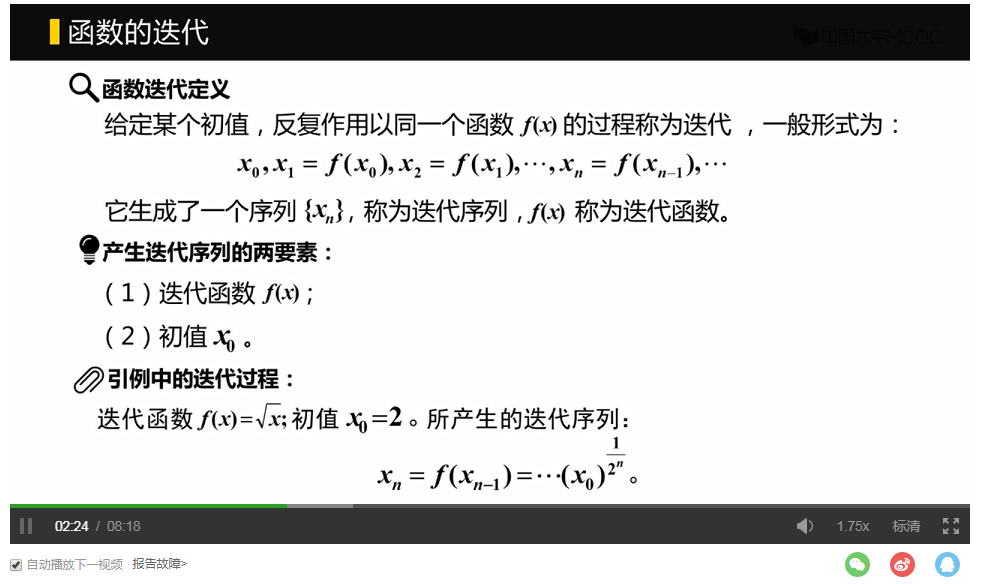定理在迭代函数连续的条件下，如果迭代序列收敛，则一定收敛于方程x=f(x)的根x*，这个根为函数的不动点。 不动点处的一阶导数小于1，则称不动点x为稳定的，若一阶导数等于0，则称不动点为超稳定，在超稳定附近，迭代过程x(n+1)=f(x(n))收敛到x*是非常快的。
用迭代法近似计算
计算三次根号2 过程分析 首先取 f(x)=2/x^2 因为f(2(1/3))=2(1/3) 在该点的导数=2>1,不满足要求
>> syms x;
>> y=diff(2/x^2);
>> subs(y,x,2^(1/3))%用值代替x
>> z=subs(y,x,2^(1/3));
>> fprintf('z = %.2f\n',z)%保留两位小数
z = -2.00

对上式恒等变形 x3=2 x3+ax2+bx=ax2+bx+2 x=(ax2+bx+2)/(x2+ax+b) 取f(x)=(ax2+bx+2)/(x2+ax+b) 令a=0,b=1,得f(x)=(x+2)/(x2+1)
对新的方程求解
>> syms x;
>> y=(x+2)/(x^2+1);
>> p=diff(y);
>> subs(p,x,2^(1/3))
>> z=subs(p,x,2^(1/3))
>> fprintf('z = %.2f\n',z)
z = -0.84

但这样收敛速度有点慢
继续变形 令a=0,b=4,得f(x)=(4x+2)/(x2+4), f(x)=(ax+b)/(cx+d) 要满足a=d,b=3c
>> y=(4*x+2)/(x^2+4);
p=diff(y);
z=subs(p,x,2^(1/3)); fprintf('z = %.2f\n',z)
z = 0.15

>> f=inline('(4*x+2)/(x^2+4)');
>> x0=12;%初值随便给一个
>> for i =1:100
x0=f(x0);
fprintf('%g,%g\n',i,x0);
end
1,0.337838
2,0.814595
3,1.12754
4,1.23501
5,1.25606
6,1.25935
7,1.25984
8,1.25991
9,1.25992
10,1.25992
11,1.25992
12,1.25992
13,1.25992
14,1.25992

迭代上式，稳定在1.25992，即为其方程的解，即三次根号2的值为1.25992验算logistics的收敛性 例如f(x)=ax(1-x),(0<=x<=1),a=3.2,x0=0.2 先编写一个Demo函数
function Demo(f,x0,N)
close all
for i =1:N
axis([50,N,0,1]);
if i>50
plot(i,f(x0),'.');
hold on ;pause(0.05);%画图加延迟
end
x0=f(x0);
end;
hold off
clear all;

命令行下敲入
Demo(@(x)3.2*x*(1-x),0.2,200)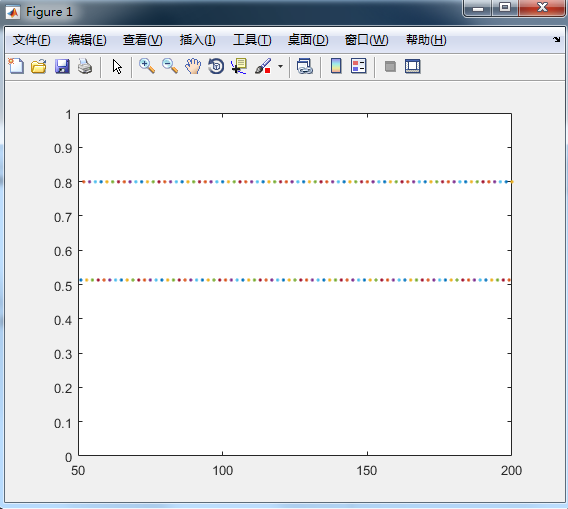可以看出形成了循环，值一直在两个值上跳动，周期为2
更改a的值，可以出现不同的收敛情况 a=3.6,x0=0.6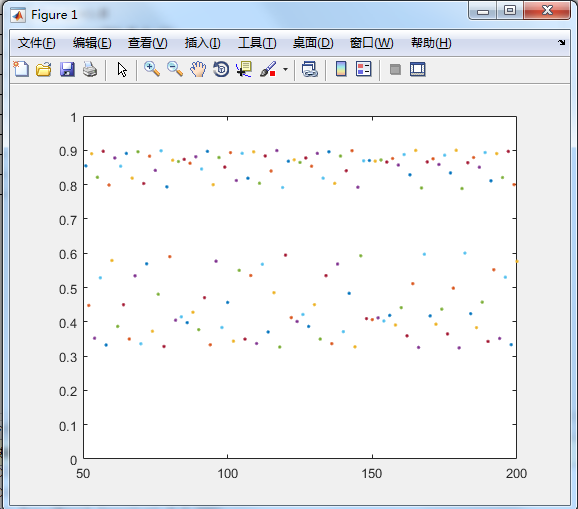出现混沌现象
logistics模型
f(x)=ax(1-x) (0<=x<=1) a>0，为logistics参数

在logistic函数中，首先取a的值为3，在（0，1）中随机取一数x。作为初值，进行迭代，共迭代300次左右，丢弃起始的100次迭代的数据，在图上绘出所有的点（a，Xn）（>100）.然后慢慢地增加a值，每增加一次，都重复前面的步骤，一直增加到a=4为止，画出最后所得到的图形。

logistic=inline('u*x*(1-x)');
for u=3.0:0.01:4
x0=rand;
for i =1:300
x0=logistic(u,x0);
if i>100
plot(u,x0,'k','linewidth',1);
hold on;
end;
end;
end;
hold off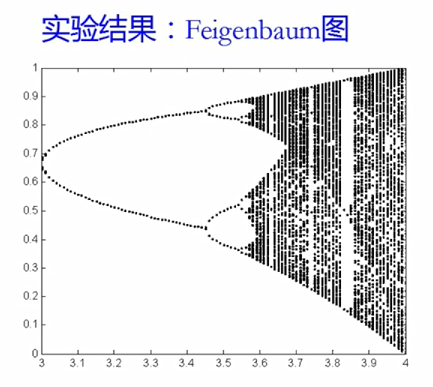形成了周期的循环 画平行于y轴的直线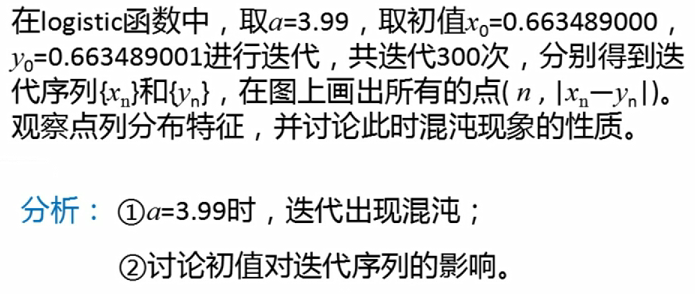初值影响很大，所以叫做蝴蝶效应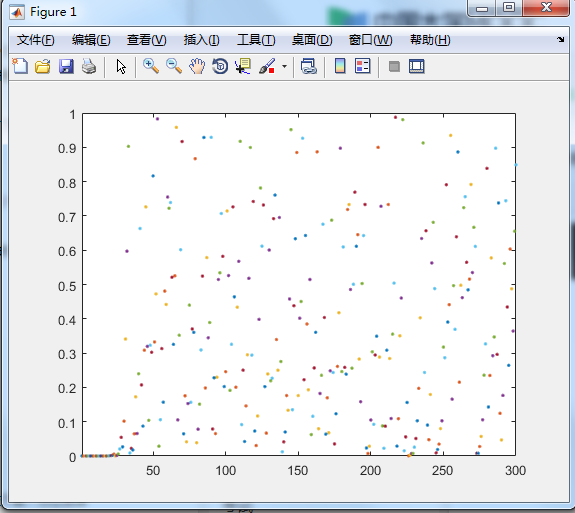close all;clear all;
f=inline('3.99*x*(1-x)');
N=300;x0_1=0.663489000;x0_2=0.663489001;
for i=1:N
axis([1,N,0,1]);
yn=abs(f(x0_1)-f(x0_2));%取两个初值函数差的绝对值
plot(i,yn,'.');
hold on;pause(0.005);
x0_1=f(x0_1);
x0_2=f(x0_2);
end;
hold off;

迭代初期，差距不大，但是后面产生混沌现象，导致结果差距很大，蝴蝶效应周期为2，可以取Feigenbaum上的值找周期
二维迭代
f=@(x,y)y-sin(x);
g=@(x)3.1-x;
xn=1.2;
yn=0;
for n=1:1000
xN=xn;
yN=yn;
xn=f(xN,yN);
yn=g(xN);
plot(xn,yn,'k*');
axis([-4,6,-3,7]);
pause(0.1);
hold on;
end
hold off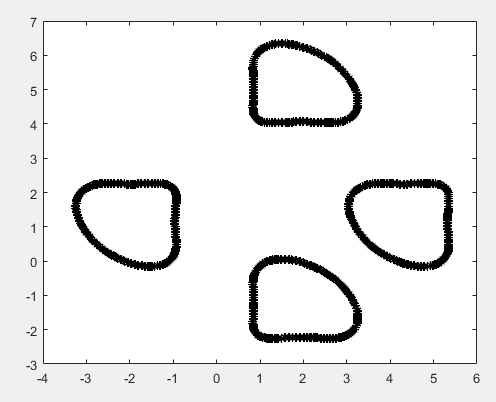小图形经过旋转、折叠后可以重合，这类图形是分形图
Martin迭代
function Martin(a,b,c,N)%N为迭代次数
f=@(x,y)(y-sign(x)*sqrt(abs(b*x-c)));%f和g称为Martin迭代
g=@(x)(a-x);
m=[0;0];
for n=1:N
m(:,n+1)=[f(m(1,n),m(2,n)),g(m(1,n))];
end
plot(m(1,:),m(2,:),'kx');
axis equal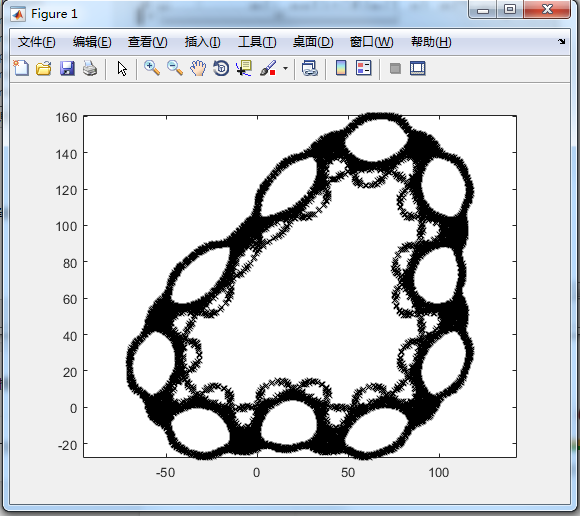局部与整体具有某种相似 但不能从二维迭代散点图中判断迭代序列是否收敛
展开全文• 方程组求解的直接法与迭代法实现 问题描述 我们的目的在于求解如下所示的方程组： 其中的A11、A12、A21、A22A_{11}、A_{12}、A_{21}、A_{22}A11​、A12​、A21​、A22​以及右端项fff以不同的的稀疏存储方式存在...
方程组求解的直接法与迭代法实现
问题描述
我们的目的在于求解如下所示的方程组：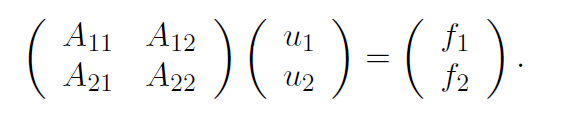其中的

A

11

、

A

12

、

A

21

、

A

22

A_{11}、A_{12}、A_{21}、A_{22}

以及右端项

f

f

以不同的的稀疏存储方式存在文件中。
我们需要完成的问题包括：
理解并描述

A

11

、

A

12

、

A

21

、

A

22

A_{11}、A_{12}、A_{21}、A_{22}

以及右端项

f

f

的数据存储方式，其中

A

11

A_{11}

是以CSR（compressed）方式存储，其余以COO（coordinate or IJ）方式存储。设计一种数据存储方式来储存具有块结构的稀疏矩阵

A

A

。写一段程序读取

A

A

的子块，并将其以设计好的结构存储为

A

A

。用MUMPS，SuperLU等方法来直接求解方程组。选择和应用一种迭代方法来求解线性方程组，并提供随着迭代步的进行的收敛情况（和直接法做比较）。基于你对这个线性系统的理解，设计一种有效的预条件子方法来加速迭代方法的收敛。
CSR和COO矩阵压缩稀疏存储方式说明
CSR：压缩稀疏行存储
N = 58324，M=99。

A

11

A_{11}

稀疏存储文件说明：
第1行3个数： 行块数（58324），列块数（58324），非零块数（385766）第2行： 每一块小矩阵的规模（边长3）第3行： 每一块的flatten方式（0表示一行一行来）第4行： 记录非零矩阵块累计数（按行）的向量的元素个数（58325）第5~58329行（58325个）： 表示非零矩阵块数目的累计值（IA），即后一个值减前一个值表示当前行的非零块个数。第58330行： 非零块的个数（385766）第58331~444096行（385766个）： 表示对应于（IA）的非零块的列标（JA，标号从0开始）第444097行： 非零元素块的元素个数综合（3471894）第444098~3915991行（3471894个）： 非零元素位置对应的值
COO：坐标稀疏存储

A

12

A_{12}

稀疏存储文件说明：
第1行3个数： 行数（174972），列数（99），非零元素（1148）第2到1149行（1148个）： 行坐标，列坐标，值

A

21

A_{21}

稀疏存储文件说明：
第1行3个数： 行数（99），列数（174972），非零元素（1100）第2到1101行（1100个）： 行坐标，列坐标，值

A

21

A_{21}

稀疏存储文件说明：
第1行3个数： 行数（99），列数（99），非零元素（99）第2到100行（99个）： 行坐标，列坐标，值

f

f

稀疏存储文件说明：
第1行： 列数（175071）第2到175072行（175071个）： 位置和值

A

A

的组装和存储
观察

A

A

的四个子块以及右端项的存储方式，我们发现，除了

A

11

A_{11}

使用CSR方式存储之外，其他部分用的都是COO的存储方式。一个简单的想法是，先把

A

11

A_{11}

装成COO存储方式，在将几个子块读入，组装成

A

A

，以COO的方式写入文件，以便备用。这个过程我用Matlab（Matlab 2014a 盗版）来实现。

A

11

A_{11}

的从CSR格式转成COO格式并保存的代码如下：
clc
clear
%% 读入数据，只读第一列
filename = 'A11.dat';
delimiter = ' ';
formatSpec = '%f%*s%*s%[^\n\r]';
fileID = fopen(filename,'r');
dataArray = textscan(fileID, formatSpec, 'Delimiter', delimiter, 'MultipleDelimsAsOne', true, 'EmptyValue' ,NaN, 'ReturnOnError', false);
fclose(fileID);
A11 = [dataArray{1:end-1}];
clearvars filename delimiter formatSpec fileID dataArray ans;
%% 处理数据，转为COO格式，并保存
n = 174972;
IA = A11(5:58329,:);
nonzero_mat_num = IA(2:end)-IA(1:end-1);%共58324个
JA = A11(58331:444096,:);
JA = JA+1;%matlab的下标从1开始
values = A11(444098:3915991,:);
row1 = [174972 174972 3471894];
submat_sparse_store_row = [];
for i=1:58324
current_num = nonzero_mat_num(i);
submat_sparse_store_row(end+1:end+current_num,1) = repmat(i,current_num,1);
end
submat_sparse_store_col = JA;
sparse_X = [];
sparse_Y = [];
sparse_values = values;
for i=1:385766
ind_row = submat_sparse_store_row(i);
ind_col = submat_sparse_store_col(i);
[x,y] = meshgrid((ind_row-1)*3+1:(ind_row)*3,(ind_col-1)*3+1:(ind_col)*3);
x_temp = x;
y_temp = y;
x_flatten = x_temp(:);
y_flatten = y_temp(:);
sparse_X(end+1:end+9,1) = x_flatten;
sparse_Y(end+1:end+9,1) = y_flatten;
end
A11_sparse = sparse(sparse_X,sparse_Y,values);
A11_sparse(2000,2000)
sum(sum((A11_sparse ~= 0)))
data = [row1;sparse_X-1 sparse_Y-1 values];
save('A11_coo.dat','data','-ascii');%ps：save方式的存储会产生误差,但是速度快咯，anyway，问题不大
%dlmwrite('A11_coo.dat',data,'delimiter','\t','precision','%0.6e');


从四个子块组装为

A

A

并以COO格式保存为A.dat的代码如下：
% 将四个系数矩阵拼成一个矩阵
clc
clear
%%
N = 58324;
M = 99;
n = 3*N;
m = M;
%%

%%
A11_h = A11_coo(1,:);
T11 = A11_coo(2:end,:);
%%
A12_h = A12(1,:);
T12 = A12(2:end,:);
T12(:,2) = T12(:,2)+n;
%%
A21_h = A21(1,:);
T21 = A21(2:end,:);
T21(:,1) = T21(:,1)+n;
%%
A22_h = A22(1,:);
T22 = A22(2:end,:);
T22(:,1) = T22(:,1) + n;
T22(:,2) = T22(:,2) + n;

%%
A_header_num = A11_h(3) + A12_h(3) + A21_h(3) + A22_h(3);
T = [T11;T12;T21;T22];
%save('A.dat','A',''-ascii','-double');

%% 写入数据，第一行和第一列和第二列以int类型写入，第三列（除第一行），以双精度类型写入。
fileID = fopen('A.dat','w');
fprintf(fileID,'%6d  %6d  %6d\r\n',A(1,1),A(1,2),A(1,3));
%fprintf('%6d  %6d  %6d\n',A(1,1),A(1,2),A(1,3));
for k=1:size(A,1)-1
fprintf(fileID,'%d  %d  %1.6e\n',A(k+1,1),A(k+1,2),A(k+1,3));
%fprintf('%d  %d  %1.6e\n',A(k+1,1),A(k+1,2),A(k+1,3));

end
fclose(fileID);

%%
% B = sparse(A(2:end,1)+1,A(2:end,2)+1,A(2:end,3));
% B_full = full(B);

Matlab的back slash方法求解方程组
\符号求解方程组
既然用了Matlab，首先，一个自然的想法就是使用Matlab的\来求解方程组。使用的代码如下：
clc
clear

%% 导入A和f的数据
f_id = fopen('f.dat','r');
fgets(f_id);
formatSpec = '%d %f';
sizef = [2 Inf];
ff = fscanf(f_id,formatSpec,sizef);
fclose(f_id);
ff = ff';
f = ff(:,2);
%%
A_h = A(1,:);
T = A(2:end,:);
%%
AA = sparse(T(1:end,1)+1,T(1:end,2)+1,T(1:end,3));
u = AA\f;
%%
u_mine = u;
save('u_mine.dat','u_mine','-ascii');

%% check result
E = u(1)-1.24456;


做个简单的计时，发现back slash求解大概需要2.655s。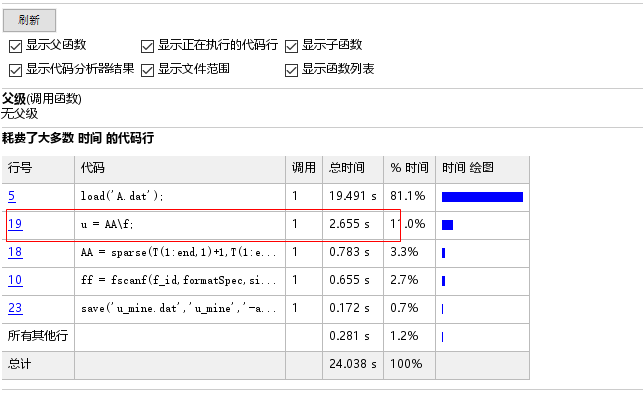求解的结果的前几个值如下：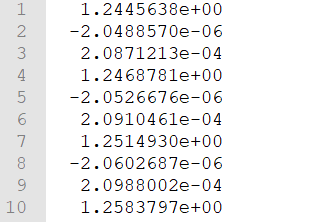我的计算机参数
自报一下家门，老电脑了，四核处理器，每个核心2.60GHz，单核每个时钟周期执行的浮点运算次数大约为25。

理

论

峰

值

速

度

=

cpu

主

频

∗

每

个

时

钟

周

期

执

行

的

浮

点

运

算

次

数

∗

核

数

理论峰值速度=\text{cpu}主频*每个时钟周期执行的浮点运算次数*核数

笔记本的峰值计算能力为256亿浮点运算/秒，具体如下：
制造商 : Intel 型号 : Intel® Core™ i5-3230M CPU @ 2.60GHz 速度 : 3.2千兆赫（GHz） 最低/最高速度/涡轮速度 : 1.2千兆赫（GHz） - 2.6千兆赫（GHz） - 3.2千兆赫（GHz） 恒速 : 2.6千兆赫（GHz） 峰值处理性能 （PPP） : 25.6数十亿浮点运算/秒（GFLOPS） 调整后的峰值性能 （APP） : 7.68WG 内核/处理器 : 2 个 线程/内核 : 2 个 类型 : 便携电脑
关于matlab内置算法的一个说明
关于matlab的backslash：对于稀疏矩阵的\(mldivide)方法求解，Matlab大多时候使用的是分解算法，如QR solver、diagonal solver、banded solver、（permuted）triangular solver、LU solver、Cholesky solver和LDLsolver等等。Matlab会自己进行条件判断，而根据矩阵情况选择合适的算法。
另外，查看了matlab的document，其对于稀疏矩阵的分解解法和迭代解法如下：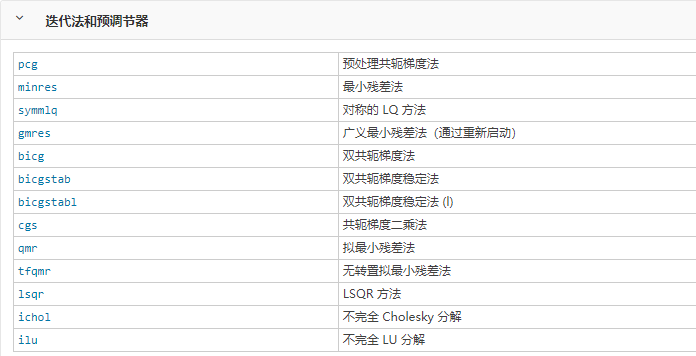对于一般的方法，有一定的适用条件：比如说pcg用于处理Hermitian正定矩阵，minres一般用于处理Hermitian矩阵。
直接法求解（MUMPS, SuperLU，etc）
Matlab没有现成的MUMPS，SuperLU的求解器。怎么办？只能使用c中的相应的库来实现。当然，也可以利用Matlab和c或者Fortran的混编，毕竟MUMPS的SuperLU的包没有matlab版本的，那么又想用Matlab来调用，这么干也是可以的。
我第一个想到的是使用所里的phg来实现，因为它有相关的接口。我在ubuntu下安装了phg及其相关的依赖，用以调试程序，等到合适的时候，再将程序放到所里的科学计算集群（LSSC-IV）上去运行。
phg的方程组求解器接口调用
编写c程序，读入Matlab生成的A.dat和已有的f.dat文件，调用phg的解法器接口，进行求解。代码如下：
#include "phg.h"
#include <string.h>
#include <math.h>
#include <stdio.h>
#include <stdlib.h>

int main(int argc, char *argv[])
{

/* Read data, including A and f. 先一次性把数据读进来，这样比较节省时间。*/
FILE *fp;
if ((fp = fopen("A.dat", "r")) == NULL)
{
printf("cannot find what you need,please check it!");
exit(0);
}
int m, n, numOfNonzero;
int i;
fscanf(fp, "%d%d%d", &m, &n, &numOfNonzero);
float *valuesOfA = (float*)malloc(sizeof(float)*numOfNonzero);
int *rows = (int*)malloc(sizeof(int)*numOfNonzero);
int *cols = (int*)malloc(sizeof(int)*numOfNonzero);
for (i = 0; i <= numOfNonzero - 1; i++)
{
fscanf(fp, "%d%d%f", &rows[i], &cols[i], &valuesOfA[i]);
}
fclose(fp);

if ((fp = fopen("f.dat", "r")) == NULL)
{
printf("cannot find what you need,please check it!");
exit(0);
}
int numOff;
fscanf(fp, "%d", &numOff);
double *valuesOff = (double*)malloc(sizeof(double)*numOff);
int *indexOff = (int*)malloc(sizeof(int)*numOff);
for (i = 0; i <= numOff - 1; i++)
{
fscanf(fp, "%d%lf", &indexOff[i], &valuesOff[i]);
//printf("%d,%0.15e\n", indexOff[i], valuesOff[i]);
}
fclose(fp);
//	printf("%d,%d,%0.6e\n", rows, cols, valuesOfA);
//	printf("%d,%0.15e\n", indexOff, valuesOff);
//	getchar();

//读如的数据存放在rows、cols、valuesOfA和indexOff以及valuesOff中。

SOLVER *solver;//创建解法器对象
MAT *A;
MAP *map;
//	MPI_Comm comm;
//	phgInitSetComm(comm);
phgInit(&argc,&argv);
//	printf("phgComm=%d",phgComm);
map = phgMapCreateSimpleMap(phgComm,-1,175071);
A = phgMapCreateMat(map,map);
int numOfNonzeroInA = 3474241;

for (i = 0; i <= numOfNonzeroInA - 1; i++)
{
int row = rows[i];
int col = cols[i];
float value = (float)valuesOfA[i];
//printf("row=%d,col=%d,value=%f\n",row,col,value);

//printf("debug: program comes here……\n");

}

solver = phgSolverMat2Solver(SOLVER_DEFAULT,A);
solver->verb = 1;

int N = 175071;

//printf("debug: program comes here……%f%f",indexOff,valuesOff);

phgVecDisassemble(solver->rhs);
for (i = 0; i <= N - 1; i++)
{
int index = indexOff[i];
float value = valuesOff[i];
value = (float)value;
//	printf("i=%dindex=%dvalue=%f",i,index,value);
//	getchar();

}

VEC *u_h;//定义VEC对象存放解
u_h = phgMapCreateVec(map,1);
//	phgVecDisassemble(u_h);

//	const char *var_name = "f_out";
//	const char *file_name = "f_out";
//	phgVecDumpMATLAB(solver->rhs,var_name,file_name);

//	var_name = "A_out";
//	file_name = "A_out";
//	phgMatDumpMATLAB(solver->mat,var_name,file_name);

phgSolverVecSolve(solver, 0, u_h);//求解
/*	const char *var_name = "u_h";
const char *file_name = "u_mine";
phgVecDumpMATLAB(u_h,var_name,file_name);
*/
return 0;

}

/*自由度对象赋值*/
/*  	//solver = phgSolverCreate(SOLVER_DEFAULT, u_h, NULL);//定义解法器
for (int i = 0; i <= numOfNonzero - 1; i++)
{
int row = rows[i];
int col = cols[i];
float value = (float)valuesOfA[i];

}
printf("debug: program comes here……\n");

for (int i = 0; i <= numOfNonzero - 1; i++)
{
int index = indexOff[i];
float value = (float)valuesOff[i];

}

phgSolverSolve(solver, TRUE, u_h, NULL);//求解
//SIMPLEX *e;
//	const FLOAT lambda[];
//	FLOAT *values;
//	phgDofEval(u_h, e, lambda, values);//不足的输入要补全。
//	phgPrintf("first value of u is : %lf\n", *values);//打印结果
phgSolverDestroy(&solver);//销毁解法器对象
*/


编译生成可执行文件后，我们来命令行传入参数来调用phg中的解法器来求解这个 方程组。phg中预装的解法器接口有： “pcg”, “bicgstab”, “gmres”, “lgmres”, “preonly”, “aps”, “2grid”, “ams”, “gather”, “asm”, “smoother”, “hypre”, “petsc”, “mumps”, “superlu”, “minres”, "diagonal"等。
按照要求，我现在想调用mumps和superlu求解。
基于phg平台的mumps方法求解
phg提供的mumps的接口，提供了以下的参数：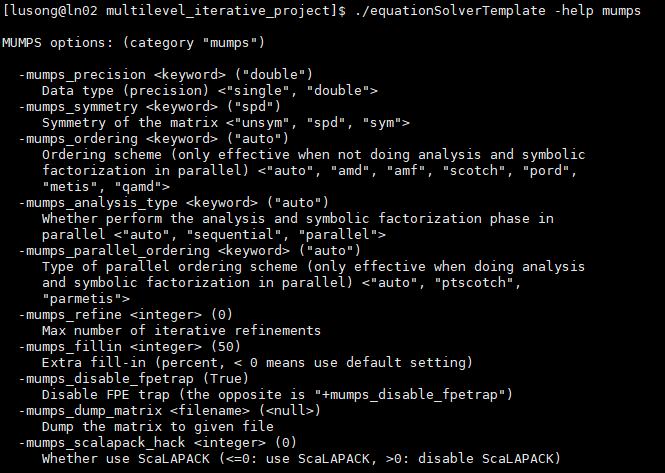在矩阵的性质中，我们给它指定非对称，其他的参数保持默认。使用如下所示命令行参数执行可执行文件：
./equationSolverTemplate -solver mumps -mumps_symmetry unsym

结果如下：
分析步：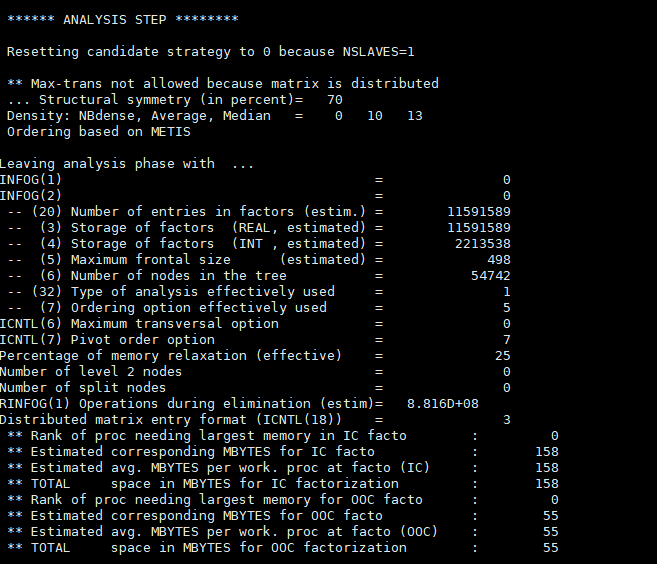分解步：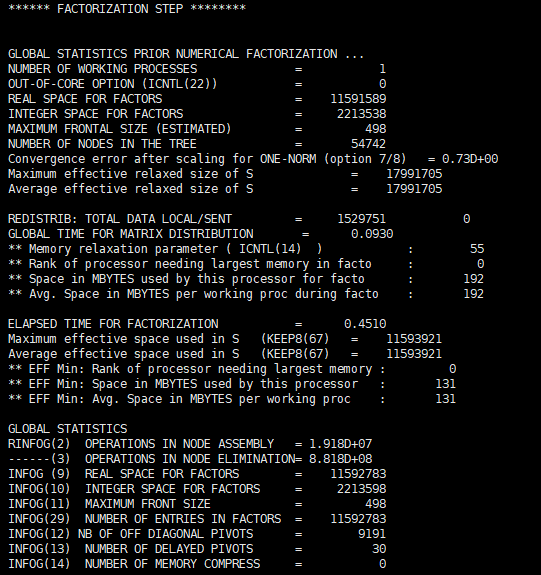求解和检查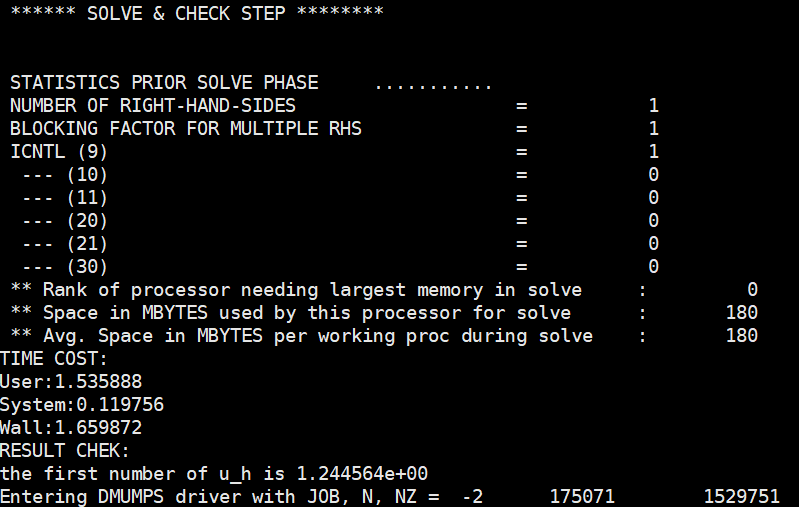可以看到，打印出来的墙上时间为1.66s，比Matlab略快一些。
基于phg平台的superlu方法求解
查看superlu的参数帮助信息如下：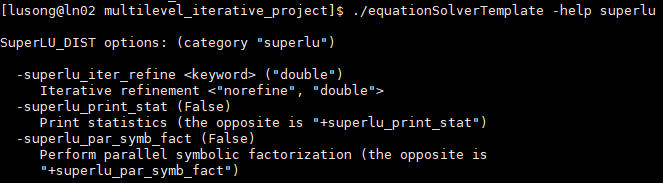我选择保持默认。使用如下命令执行可执行程序。
./equationSolverTemplate -solver superlu

结果如下：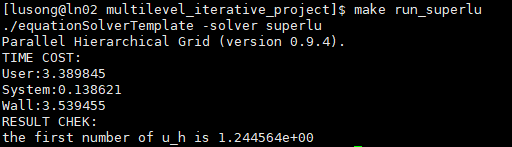消耗的墙上时间约为3.54s，比MUMPS方法略慢一些。
Anyway，我们由直接法，不管是Matlab的back slash还是MUMPS抑或是SUPER LU，我们用直接法得到了一个可以在迭代法中作为标准的近似真解。我们可以把这个解保存下来，下面再使用这个解来作为迭代法收敛性分析的一个标准。
迭代方法设计与求解

A

A

矩阵性态的观察
使用Matlab的spy函数，我们来观察一下矩阵

A

A

的分布，结果如下：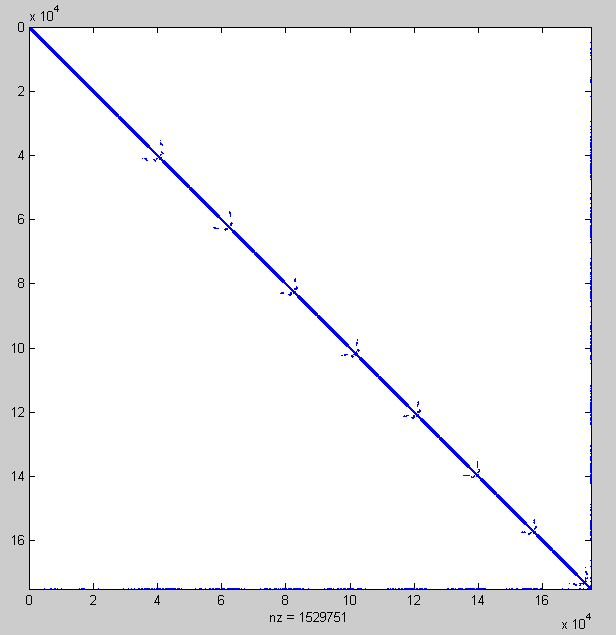看起来是个对角矩阵，而且很稀疏。我们再通过sum(sum((A - A')))判断，发现

A

A

并不是对称的，只不过是在外形上看起来有些对称（事实上，在外形上也不是对称的）。进一步，我们通过[r,p]=chol(A)判定，它并不是正定的（非对称意义下的正定）。
所以，这是一个非零元素基本上都集中在对角线以及两条边上的没有特别好的性质的稀疏矩阵。
方法的选择
我们可以设计和使用多层迭代方法来求解。时间的原因，我们依然调用别人已经写好的包，而不自己动手完成这个过程。能使用的包就比较多了，比如由张晨松老师等人编写维护的FASP的包，下载地址，另外还有Matlab内置的共轭梯度，残差法等等。
因为这个矩阵的在性质上，好像除了看起来有点对称以及稀疏之外，没有太好的性质。所以，我想采用GMRES方法求解。GMRES中文叫广义极小剩余法，它是上世纪末到本世界初被人们认为是求解大型稀疏非对称线性方程组的最有效的方法之一。Matlab有gmres内置函数，基于重启动的gmres方法。我想用的依然是phg的内置的gmres算法。
为了加快的速度，我用的是预条件子的GMRES方法。phg中gmres solver的参数如下所示。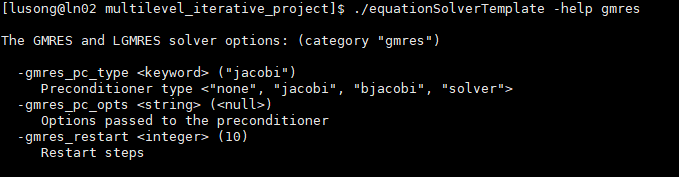预条件子的选择，使用GMRES，我的预条件子选择了两个：一个是MUMPS作为预条件子，另一个是ASM（加性 Schwarz作为预条件子），并且在ASM预条件子中使用单精度的稀疏直接求解器MUMPS求解子区域问题。
基于MUMPS预优的GMRES方法
指定用单精度的MUMPS解法器做为GMRES的预条件子。我在 Makefile中定义的命令行参数如下：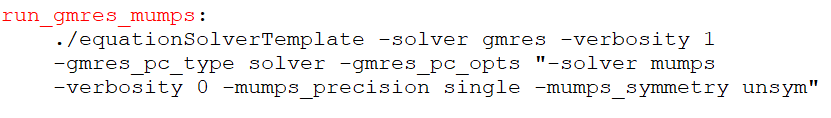上图中，gmres_pc_type 中的 “solver” 参数表示在 GMRES 每步迭代中调用另一个解法器做为预条 件子，而 -gmres_pc_opts 选项则用来设定预条件子解法器及参数。
运行的结果如下：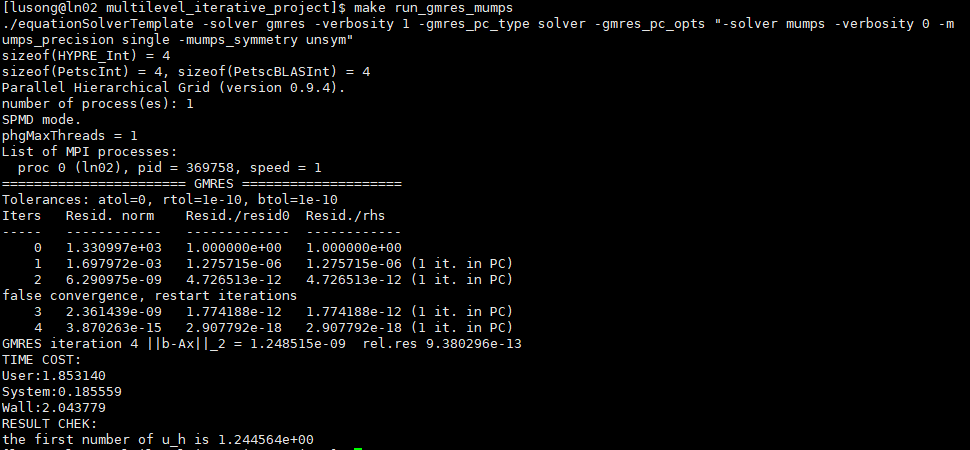使用单个进程，设定atol=0, rtol=1e-10, btol=1e-10。从结果中可以看出，迭代2次之后，有一次重启动。迭代4次残差相对值达到了-18次方量级的。墙上时间消耗时间为2.04s。收敛速度是非常快的。这是在最外层的迭代，内层表现我们就不往里看了。
基于ASM预优（ASM中使用MUMPS求解子区域问题）的GMRES方法
指定使用 GMRES 迭代、ASM (加性 Schwarz)预条件子，并且在 ASM 预条件子中使用单精度的稀疏直接求解器 MUMPS 求解子区域问题。
在Makefile中定义的参数列表如下：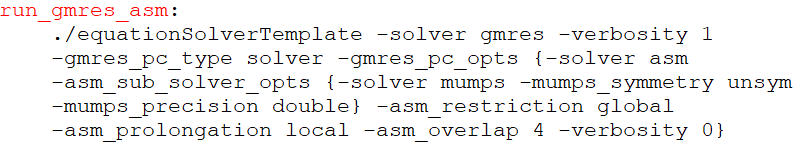结果如下：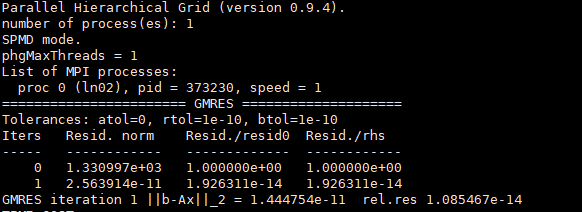经过一次的迭代（最外层的求解器），误差（相对残差）达到了e-14量级的。墙上时间消耗为1.81s。
使用Matlab的gmres内置函数求解
我们考虑一种比较简单的情况，即使用不完全LU预优分解，不采用重启动技术，我们来看看它的收敛表现。
这一次，我们不用残差来衡量误差，而使用直接法得到的解作为一个参考标准。写一段简单的matlab脚本如下，用来调用matlab的gmres内置函数。
clc
clear
%% 导入A，f和真解u
%A_h = A(1,:);
T = A(2:end,:);
AA = sparse(T(1:end,1)+1,T(1:end,2)+1,T(1:end,3));
A = AA;

f_id = fopen('f.dat','r');
fgets(f_id);
formatSpec = '%d %f';
sizef = [2 Inf];
ff = fscanf(f_id,formatSpec,sizef);
fclose(f_id);
ff = ff';
f = ff(:,2);

u = u_mine;

clear AA f_id ff formatSpec sizef T u_id uu;
%%
[L,U] = ilu(A,struct('type','ilutp','droptol',1e-6));
l = length(f);
tol = 1e-12;
maxit = 20;
b = f;

x0 = zeros(l,1);
errors = [norm(x0 - u)/norm(u);];
for i = 1:maxit
[x0,fl0,rr0,it0,rv0] = gmres(A,b,1,tol,1,L,U,x0);
errors(end+1) = norm(x0 - u)/norm(u);
end
st = 4;
semilogy(st:maxit,errors(st+1:end),'-o');
xlabel('Iteration number');
ylabel('Relative Error');

结果如下：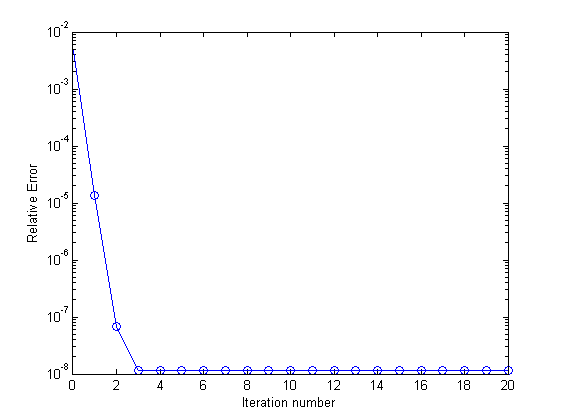这里定义的误差为

Error

=

∣

∣

u

−

u

h

∣

∣

2

/

∣

∣

u

∣

∣

2

\text{Error} = ||u-u_h||_2/||u||_2

。这里的u，用的是直接法得出来的解。可以看得出来，使用不完全LU分解做预优的GMRES方法，只要两三步就达到比较高的精度了。也容易看出，迭代到第4步，误差就降低到了e-8量级的。另外，这个过程也不是单调的，在第5步到第6步，误差有一个非常微小的升高。
展开全文SUPERLU 方程组求解 稀疏矩阵
• matlab迭代法程序代码量化宏观经济学，2018-2019 UAB经济学博士学位第二年课程 介绍 我们的课程分为两个部分。 A部分是从基本数值方法到使用VFI，PFI解决代表代理模型（永久收入，生命周期）的方法； 最终进入市场不...
• 北航计算机学院-计算机组成原理课程设计-2020秋，PreProject-Logisim-Logisim组合逻辑电路-斐波那契数列问题(简单迭代法+矩阵乘法的快速幂)。 北航计算机学院的计算机组成原理课程设计，是高度实践性的专业课程，...
北航计算机学院-计算机组成原理课程设计-2020秋
PreProject-Logisim-斐波那契数列问题(简单迭代法+矩阵乘法的快速幂)

从本节开始，课程组给出的教程中增添了很多视频讲解。为了避免侵权，本系列博客将不会搬运课程组的视频讲解，而对于文字讲解也会相应地加以调整，重点在于根据笔者自己的理解给出习题的解析。因此带来的讲解不到位敬请见谅。

文章目录
北航计算机学院-计算机组成原理课程设计-2020秋PreProject-Logisim-斐波那契数列问题(简单迭代法+矩阵乘法的快速幂)斐波那契数列问题的简单迭代法斐波那契数列-矩阵乘法快速幂法斐波那契数与矩阵乘法快速幂快速幂的迭代表达Logisim电路实现

斐波那契数列问题的简单迭代法
提交要求
使用Logisim搭建一个根据输入序号x计算对应序号斐波那契数fib[x]的电路（输入序号0对应输出数0，输入序号1对应输出数1，输入序号2对应输出数1，以此类推）并提交。
输入： N（3bit）输出： Nth（4bit）文件内模块名: main测试要求：每次给定一个固定输入保持不变，电路在64个周期内计算出结果并稳定输出，在结果未计算出之前输出端口输出0请使用时序逻辑完成本题目。未按照要求（或采用捷径）完成并提交通过的同学，请重新提交。
提到斐波那契数列，想必大家小学二年级就已经学过了经典的1, 1, 2, 3, 5, 8, …，在程序设计入门的时候大家也一定写过斐波那契数的实现代码，最直观地，我们通过定义可以写出递归版本的斐波那契数（以下展示C语言版本）：
int fib(int n) {
if (n <= 2) return 1; // fib(1) = fib(2) = 1
return fib(n - 1) + fib(n - 2);
}

但是放在本题，是无法用这样的思路直接实现电路的，因为递归实质上是操作系统在不断索取硬件资源，我们无法让电路实现递归式的栈增长，因此需要考虑迭代的方法：
int fib(int n) {
int prev = 1, now = 0; // fib(-1) = 1, fib(0) = 0;
for (int i = 0; i < n; i++) {
now = now + prev;
prev = now - prev;
}
return now;
}

我们有没有可能用电路实现循环控制呢？答案是肯定的。我们可以使用寄存器来存储变量的值，然后通过其他运算器件在相邻时钟上升沿之间改变寄存器的输入，更新寄存器的值，最后通过计数器确定运算的次数进行输出即可。

题外话，题目所述的“必须采用时序逻辑电路”、“禁止走捷径”，走捷径的方法是什么呢？很容易，输入只有3位，我们将输入范围内的斐波那契数全部存储在RAM里，根据输入读出该值即可（大家不要采用这种方法。

下面的介绍将自顶向下地描述电路构建成果。首先电路在顶层之下划分出“计数逻辑”和“计算逻辑”两个子电路，“计算逻辑”是整个电路的核心，其功能主要是计算出不断更新的fib(n)的值；“计数逻辑”是电路正确输出的保障，其主要功能是给出电路何时应当输出答案的信号。两者配合达到的效果是：一端不断更新fib(n)数列，另一端在合适的时候输出数列的当前值，就像是列车的发动机只负责提供向前的动力，而控制系统掌管列车何时到站停下。顶层电路的设计如下图所示。 按照题目要求，左侧三位输入是待求fib(n)中的n，右侧四位输出是fib(n)的值，在计算得到结果之前输出0，得到正确结果之后稳定输出fib(n)。为了增强电路的可读性，我在设计中尽可能多地使用了Tunnel来说明每一根信号的功能。左下方的reset信号是在设计过程中为了方便调试添加的复位信号，在调试时将该信号设置为1则整个电路回到初始状态，子电路中所有计数器和寄存器的值全部清零。该信号单纯为了调试方便，和整体的功能没有直接关系，可以直接删除。该信号接入了两个子电路，下文的子电路介绍中不再阐述。
上面已经提到，计数逻辑子电路主要功能是给出电路何时应当输出答案的信号，当计数逻辑右侧的输出信号output_signal为0时，右侧多路选择器选择常数0，输出保持为0，而当output_signal为1时，将输出计算逻辑子电路的输出值，也就是计算得到的正确答案。上文同样提到，计算逻辑将会不断地更新fib(n)数列，而并不关心输出值如何稳定保持，因此output_signal信号会被接入计算逻辑，在应当输出的时候，通过寄存器enable端口冻结计算逻辑中寄存器的值，让计算逻辑的输出一直保持为正确输出，这样就实现了“得到结果前一直输出0，得到结果后该结果稳定输出”的效果。
计算逻辑子电路的设计如下图所示。 首先观察中部两个寄存器的设计，回顾在前面的分析中我们得到的fib(n)算法，其中循环体里用到了两个变量prev和now，分别表示数列当前值和上一个值，初始时要对两个值赋初值，分别表示fib(-1) = 1, fib(0) = 0，随后开始的循环中now = now + prev; prev = now - prev。根据这样的思路，我们使用两个寄存器来分别存储prev和now的值，在每一次循环中，now寄存器应当被更新为now + prev，那么只需将两个寄存器输出端口求和接入now寄存器即可。而对于prev寄存器，只需要直接接入now的输出值即可，而不是计算now - prev，这是由于我们书写在C语言中的算法，其语句是顺序执行的，在now被更新后就必须使用now - prev的值作为新的prev值，而在此处prev和now是同时更新的。换句话说，正确的算法是prev’ = now’ - prev = now，因此直接接入now的输出即可。根据这样的特点我们也会发现，原本每个循环需要执行两次的循环体，在电路实现中只需要一个时钟周期就可以完成。
真正的计算很简单，但还有一个棘手的问题，就是如何优雅地为寄存器赋初值。考虑到电路的应用性，我们不应当每次手动为寄存器赋值为1，因此需要考虑设计一种机制，让第一个时钟周期给prev寄存器赋值为1，now寄存器赋值为0，而在后面的时钟周期根据上面一段叙述的逻辑改变两者的值。在此处我采用的解决办法是使用一个1位计数器，并设置计数器达到上限后"stay at value"，保持最大值不变（具体设置方法如下图），这样一来该计数器只有第一个时钟周期输出0，后面一直输出1，而此时将其输出作为选择信号接入多路选择器，就实现了prev寄存器第一个周期赋初值，后面的周期赋值为now。而对于now寄存器，第一个周期now和prev之和本身就是0，无需特殊处理。最终电路始终输出now的值即可。在顶层电路的描述中我们提到，为了保证得到正确答案后稳定输出，我们采用的方式是将计数逻辑输出信号接入计算逻辑，也就是左下方的freeze信号，当该信号为1时，代表外部提醒：已经得到正确答案了，请冻结输出。此时将freeze信号取反接入两个寄存器的enable端即可，没有得到正确答案前寄存器正常工作，一旦得到冻结信号，寄存器停止工作，稳定输出当前值。
分析过后我们考虑电路的计算特点：在第一个周期为寄存器赋初值，其后的每一个周期输出当前值，那么计算fib(n)就需要(n+1)个时钟周期，具体来说就是(n+1)个时钟上升沿，这条结论就指导了我们下一步设计计数逻辑，应当在(n+1)个时钟上升沿过后再发出输出指令。就像列车的目标是前进100公里，我们只有知道了发动机每小时让列车前进例如50公里，才能够设计控制系统，让列车在2小时之后停下。
**计数逻辑子电路如下图所示。**有了上面计算逻辑的分析和构建，下面我们很容易设计计数逻辑：让该子电路在第(n+1)个时钟上升沿之前都输出0，代表着此时还未计算出结果，在第(n+1)个上升沿之后输出1，代表此时已得到正确结果，计算逻辑冻结并输出当前值。下面的电路中只需要使用counter，当计数器的值小于等于输入n时均输出0，随后输出1即可，注意此处的counter也需要设置到达最大值时"stay at value"。至此整个电路已经构建完毕了。本设计更注重的是模块化的思路，而不在乎一两个元件的得失，例如我们完全可以将计数逻辑中counter的值作为一路信号接入计算逻辑，可以省去计算逻辑中赋初值所用的counter，但是本设计并没有这么做，而是让两个逻辑子电路尽可能地解耦。当然，这也只是笔者个人想法，应当有更精巧的思路值得大家探索。笔者认为，不管是软件还是硬件设计，有时候工程化模块化的设计布局，工整良好的可读性，可维护和可扩展性，是比小技巧更值得我们注意的关键点。

斐波那契数列-矩阵乘法快速幂法
黄小板同学暗中观察了公司负责人很久，觉得他搭建的电路性能实在太差，他提出只需要64个周期就能计算出32位无符号整数能表示的最大数位置上的斐波那契数的（最后32bit），在完成搭建这样的电路后，公司负责人五体投地，宣布给黄小板开出了东门烤串无限量供应的实习工资，从此黄小板每日吃串，终于吃成了黄老板…
那么，这个电路是什么样子的呢？
注意：这道题是一个对你的挑战，需要一定的算法和工程能力，请谨慎思考，大胆尝试！
提交要求
使用Logisim搭建一个根据输入序号x计算对应序号斐波那契数fib[x]的电路（输入序号0对应输出数0，输入序号1对应输出数1，输入序号2对应输出数1，以此类推）并提交。
输入： N（32bit无符号数）输出： Nth（32bit无符号数，表示第N个斐波那契数）文件内模块名: main测试要求：在64个周期内计算出结果并稳定输出，在结果未计算出之前输出端口输出0。HINT：矩阵乘法的快速幂
观察此题，和上题一样都是计算斐波那契数，区别仅仅在于输入从3位变成了32位无符号整数，而时间要求仍然是64个时钟周期。我们试想，如果仍用上面的电路会如何？该电路计算fib(n)需要(n+1)个时钟周期，此时输入变为32位，那么最大输入则为232-1，需要232个时钟周期，这远远超过了64个。换言之，想要在64个时钟周期内计算出32位无符号整数输入，算法的复杂度应当为O(logn)级的。有没有可能实现这样的算法呢？
题目的最后一句给出HINT：矩阵乘法的快速幂。小伙伴们立马懵逼了，矩阵乘法和斐波那契数有啥关系呀，还快速幂？这一概念我们拆开来看，先是矩阵乘法，后是快速幂。
斐波那契数与矩阵乘法
斐波那契数的通项我们都知道，

f

i

b

(

n

)

=

f

i

b

(

n

−

1

)

+

f

i

b

(

n

−

2

)

fib(n) = fib(n-1) + fib(n-2)

，但现在我们多写一项，写成如下形式：

f

i

b

(

n

)

=

1

∗

f

i

b

(

n

−

1

)

+

1

∗

f

i

b

(

n

−

2

)

f

i

b

(

n

−

1

)

=

1

∗

f

i

b

(

n

−

1

)

+

0

∗

f

i

b

(

n

−

2

)

fib(n) = 1 * fib(n-1) + 1 * fib(n-2)\\ fib(n-1) = 1 * fib(n-1) + 0 * fib(n-2)

为什么要多写一次没用的第二项呢？受上面一道题的启发，如果要将原本复杂度为O(2n)的算法，我们多使用了一个变量空间，同时更新fib(n)和fib(n-1)，将上一个斐波那契数的值保留下来，是一种“空间换时间”的策略，那么此时我们所做的操作和上一道题是完全一样的，想要实现同时更新fib(n)和fib(n-1)。换言之，在“矩阵乘法”部分，我们相对上一题还没有实现任何优化，而仅仅是改换了计算形式。

接着，根据我们刚刚学过的线性代数，引入矩阵乘法如下：

(

1

1

1

0

)

∗

(

f

i

b

(

n

−

1

)

f

i

b

(

n

−

2

)

)

=

(

1

∗

f

i

b

(

n

−

1

)

+

1

∗

f

i

b

(

n

−

2

)

1

∗

f

i

b

(

n

−

1

)

+

0

∗

f

i

b

(

n

−

2

)

)

=

(

f

i

b

(

n

)

f

i

b

(

n

−

1

)

)

\begin{pmatrix} 1 & 1 \\ 1 & 0 \end{pmatrix} * \begin{pmatrix} fib(n-1) \\ fib(n-2) \end{pmatrix} = \begin{pmatrix} 1 * fib(n-1) + 1 * fib(n-2) \\ 1 * fib(n-1) + 0 * fib(n-2) \end{pmatrix} = \begin{pmatrix} fib(n) \\ fib(n-1) \end{pmatrix}

由此我们得到了全新的，一次更新两项的递推公式：

(

1

1

1

0

)

∗

(

f

i

b

(

n

−

1

)

f

i

b

(

n

−

2

)

)

=

(

f

i

b

(

n

)

f

i

b

(

n

−

1

)

)

\begin{pmatrix} 1 & 1 \\ 1 & 0 \end{pmatrix} * \begin{pmatrix} fib(n-1) \\ fib(n-2) \end{pmatrix} = \begin{pmatrix} fib(n) \\ fib(n-1) \end{pmatrix}

记

(

f

i

b

(

n

)

f

i

b

(

n

−

1

)

)

=

F

I

B

(

n

)

,

(

1

1

1

0

)

=

A

,

记 \begin{pmatrix} fib(n) \\ fib(n-1) \end{pmatrix} = FIB(n), \begin{pmatrix} 1 & 1 \\ 1 & 0 \end{pmatrix} = A,

则

有

F

I

B

(

n

)

=

A

∗

F

I

B

(

n

−

1

)

,

其

中

F

I

B

(

0

)

=

(

f

i

b

(

0

)

f

i

b

(

−

1

)

)

=

(

0

1

)

则有\space FIB(n) = A * FIB(n-1), \space 其中 \space FIB(0) = \begin{pmatrix} fib(0) \\ fib(-1) \end{pmatrix} = \begin{pmatrix} 0 \\ 1 \end{pmatrix}

我们惊喜地发现，这一版本的递推公式不仅可以一次更新两项，还成功地将加法转化为乘法，将二阶递推式转化为一阶递推式，将加和关系转化为等比数列。又根据矩阵乘法满足交换律，该递推式容易得到通项如下：

F

I

B

(

n

)

=

A

n

∗

F

I

B

(

0

)

=

(

1

1

1

0

)

n

∗

(

0

1

)

FIB(n) = A^n * FIB(0) = \begin{pmatrix} 1 & 1 \\ 1 & 0 \end{pmatrix}^n * \begin{pmatrix} 0 \\ 1 \end{pmatrix}

根据线性代数学过的知识，2*2的矩阵不管多少次乘方，其结果都还是2*2的矩阵，

记

(

1

1

1

0

)

n

=

(

a

b

c

d

)

,

则

F

I

B

(

n

)

=

(

f

i

b

(

n

)

f

i

b

(

n

−

1

)

)

=

(

1

1

1

0

)

n

∗

(

0

1

)

=

(

a

∗

0

+

b

∗

1

c

∗

0

+

d

∗

1

)

=

(

b

d

)

记 \space \begin{pmatrix} 1 & 1 \\ 1 & 0 \end{pmatrix}^n = \begin{pmatrix} a & b \\ c & d \end{pmatrix}, \space 则 \space FIB(n)= \begin{pmatrix} fib(n) \\ fib(n-1) \end{pmatrix} = \begin{pmatrix} 1 & 1 \\ 1 & 0 \end{pmatrix}^n * \begin{pmatrix} 0 \\ 1 \end{pmatrix} = \begin{pmatrix} a * 0 + b * 1 \\ c * 0 + d * 1 \end{pmatrix} = \begin{pmatrix} b \\ d \end{pmatrix}

也就是说，我们所关心的fib(n)，只是矩阵A的n次乘方，这一2*2矩阵的右上角的数。
有了以上的分析，我们的目标很明确：计算A的n次乘方即可。但小伙伴们会发现，这一顿操作猛如虎，结果好像并没有改进算法的时间复杂度：矩阵的n次乘方还是要计算n次，毕竟Logisim并没有内置一次计算某数n次方的元件，更不用说矩阵还同时涉及乘法和加法。因此有了矩阵乘法的改写还远远不够，下面我们引入快速幂。
快速幂
先做一个引入的思考：假设每次只能做两个数之间加减乘除的运算，对于一个整数a，要求an，应当如何设计算法？
最简单直观的算法：引入一个变量s赋初值为1，使用n次循环，每次为s乘上一个a，最终s = an，如下：
int power(int a, int n) { // 计算 a^n 的值
int s = 1;
for (int i = 0; i < n; i++)
s *= a;
return s;
}

这种算法的时间复杂度显然是O(n)，有没有什么办法更快地计算a的n次幂呢？我们思考，假设我们已经计算得到了22=4，那么要求24就无需再花两次的时间计算23 = 22 * 2, 24 = 23 * 2，可以直接进行计算：24 = 22 * 22，只需要一次计算。更进一步地，我们会发现在每次只能做两个数的乘法的条件下，相乘的两数尽可能大，就可以尽可能快地接近待求结果。例如：

a

17

=

(

a

8

∗

a

8

)

∗

a

=

(

(

a

4

∗

a

4

)

2

)

∗

a

=

(

(

a

2

∗

a

2

)

2

)

2

∗

a

=

(

(

(

a

2

)

2

)

2

)

2

∗

a

a^{17} = (a^8 * a^8) * a = ((a^4 * a^4)^2)*a=((a^2*a^2)^2)^2*a=(((a^2)^2)^2)^2*a

将高次幂向下拆解的过程中，每次尽可能使用最大的两数平方来逼近高次幂，这样原本需要计算17次的a17现在只需要计算5次。在分析中我们还可以发现，当前幂次如果是偶数，就可以直接分解为两个数的平方：a2n = (an)2，需要一次计算；而如果当前幂次为奇数，则必须分解为两个数的平方再乘底数：a2n+1 = (an)2 * a，需要两次计算（一次算两数平方，一次算平方后再与底数相乘），根据这样的规律可以得到递推关系如下：

p

o

w

e

r

(

a

,

n

)

=

{

p

o

w

e

r

(

a

,

n

/

2

)

∗

p

o

w

e

r

(

a

,

n

/

2

)

n为偶数，且 n > 0

p

o

w

e

r

(

a

,

[

n

/

2

]

)

∗

p

o

w

e

r

(

a

,

[

n

/

2

]

)

∗

a

n为奇数，[x]表示不超过x的最大整数

1

n = 0

power(a, n)= \begin{cases} power(a, n/2) * power(a, n/2)& \text{n为偶数，且 n > 0}\\ power(a, [n/2]) * power(a, [n/2]) * a& \text{n为奇数，[x]表示不超过x的最大整数}\\ 1 & \text{n = 0} \end{cases}

根据递推关系就可以直接写出如下的递归算法（逻辑与(n&1)用以快速判定奇偶，内联函数用以减少power()的调用，逻辑右移(n >> 1)用以快速实现n / 2取整）：
inline int sqr(int a) {
return a * a;
}

int power(int a, int n) {
if (n == 0) return 1;
return (n & 1) ? sqr(power(a, n >> 1)) * a : sqr(power(a, n >> 1));
}

分析这一递归算法，每一递归实例唯一地调用下一个递归实例，每次调用时递归变量a不变，n减小为原来的一半，直到n = 0，因此递归深度应当为log2n；而在每个递归实例之中，n为偶数时需要1次计算（一次平方即可），n为奇数时需要2次计算（一次算平方，一次算平方的结果再乘底数a），因此整体的算法最多计算2log2n次（每次遇到的n都是奇数），最少计算log2n次（每次遇到的n都是偶数），时间复杂度O(logn)，成功地实现了将O(n)降为O(logn)。这便是快速幂的思想，其本质是一种分治算法。
这种快速幂算法能否移植到矩阵乘法上呢？也就是说，上文中的整数a能否替换为矩阵A呢？答案是肯定的，因为矩阵乘法满足结合律，并且同阶的方阵相乘后，其结果仍是与原来阶数相同的方阵。只需要将上面算法中的整数乘法全部替换为矩阵阵法，就得到了矩阵乘法的快速幂算法。
快速幂的迭代表达
当我们欣喜地发现已经找到O(logn)计算n次幂的算法后，现实的问题又摆在了眼前：硬件设计中我们无法使用递归。因此与上一题O(n)算法一样，我们需要将递归改写为迭代，通过寄存器的更新和外部计数控制来实现循环。如果你的数据结构功底扎实，将递归改写为迭代应该不是什么困难的事，你可以跳过本节的讲解。
本题将递归改为迭代主要的难点在于，递归的过程中有针对当前递归值的判断：当前的n若为奇数，则取

(

a

n

/

2

)

2

∗

a

(a^{n/2})^2*a

，若为偶数则取

(

a

n

/

2

)

2

(a^{n/2})^2

，在反向后自底向上迭代的过程中，无法确定当前的i是由奇数n还是偶数n递归而来。事实上大部分有难度的递归改迭代，其难点都在于反向后的计算逻辑和正向的有较大差别，需要分析计算的本质。
针对递归中的奇偶分类判断，我们做如下的思考：先从极端情况考虑，什么样的数n每次递归实例都是偶数？什么样的数n每次递归实例都是奇数？第一个问题容易回答，如果n是2的幂，n = 2k，那么每次n >> 1之后仍旧是偶数，这意味着n的二进制展开除了最高位之外每一位都是0（这样右移一位之后最低位是0，仍是偶数）；有了第一个问题，第二个问题也有了思路：如果每次n >> 1后都是奇数，这意味着n的二进制每一位都是1（这样右移一位之后最低位还是1，仍是奇数）。推广到一般情况：任意数n在递归过程中有多少次奇数多少次偶数？这取决于n的二进制展开中有几个1和几个0，1的个数就是递归实例为奇数的个数。
有了这样的启发，我们可以重新思考快速幂的计算过程：

a

13

=

a

(

1101

)

b

=

a

(

1000

)

b

+

(

0100

)

b

+

(

0001

)

b

=

a

8

+

4

+

1

=

a

8

∗

a

4

∗

a

1

=

(

(

a

2

)

2

)

2

∗

(

a

2

)

2

∗

a

a^{13} = a^{(1101)_b} = a^{(1000)_b+(0100)_b+(0001)_b} = a^{8+4+1}\\ = a^8 * a^4 * a^1 = ((a^2)^2)^2 * (a^2)^2 * a

在上面的例子中，假设幂次n本身是2的幂，其二进制展开除了最高位都是0，那么最终形式里不会有多余的乘号（只会是a的log2n次平方），计算log2n次平方即结束。该例子中的13次方，二进制展开后有3个1，最终形式里有3个乘号，多算3次，这与递归方法中的特点相吻合。重要的是，以这种形式展开后，计算过程是自底向上的：我们想象一个乘子m，其初始值为a，每次更新让m变为自身的平方（1次计算），同时扫描幂次n的二进制展开位，当扫描到当前位是1时，意味着“需要多一次乘法了”，就将当前乘子的值乘入最终结果（多1次计算）。仍旧分析上面的例子，初始乘子为a，扫描到13最低位是1，此时将乘子a乘入最终结果（最终形式中最后一项"a"）；第二轮乘子更新为自身的平方a2，扫描到13二进制展开次低位为0，无需将乘子乘入最终结果；第三轮乘子更新为自身的平方(a2)2，扫描到13二进制展开次高位为1，将乘子乘入最终结果（最终形式中的第二项"(a2)2"）；第四轮乘子更新为自身的平方((a2)2)2，扫描到13二进制展开最高位为1，将乘子乘入最终结果（最终形式中的第一项"((a2)2)2"），四轮结束后算法结束，得到了最终形式的正确结果。
int power(int a, int n) {
int s = 1; // 存储最终结果
while (n > 0) { // 依次扫描 n 的每个二进制位直到 n = 0
if (n & 1) s *= a; // 如果当前二进制位为 1，则将乘子当前值乘入最终结果
a *= a; // 乘子更新为自身的平方
n >>= 1; // n 向右移位，扫描下一位
}
return s;
}

Logisim电路实现
有了上面大篇幅的算法理论分析，接下来就是在电路中实践了——将上面的C语言算法转化为Logisim电路。如上图，本次工程延续了上一道题中，计算逻辑和计数逻辑解耦的基本结构，但由于本题设计矩阵乘法，存储和计算所用的元件比较多，根据模块化设计思想拆分出了较多的子电路，分别设计和调试以实现高效地开发。本节的讲解我们首先介绍作为支持功能的几个子电路。上图展示了寄存器矩阵子电路。由于计算逻辑中矩阵乘法需要以整个矩阵的形式一次存储四个数，摆放过多的寄存器显得臃肿杂乱，因此将这部分单独拆开为子电路，其功能和结构非常明确，4个32位寄存器，其输入输出接口和传统寄存器完全一致。上图展示了矩阵乘法子电路。在前文的算法分析中可以看出，我们需要在计算逻辑中作2*2矩阵的乘法，因此讲乘法过程单独拆开作为子电路。2*2矩阵乘法的计算也相对简单，套用公式用元件计算相应结果的输出即可。上图展示了矩阵MUX子电路。该子电路的构建是由于计算逻辑中，我们会遇到需要选择两个矩阵中的一个进行运算的情况（例如当前n的末位为1时乘入乘子的值，为0则乘单位阵），因此为了方便一次选择一组四个数，我们将四个多路选择器组合单独设计为子电路，由一路1位信号选择两个矩阵的其中一个作为输出。上图展示了矩阵初始值子电路和单位阵子电路。没错，我将常数单独封装为子电路应用于设计中，这可能并不符合某些设计原则，但可以在上层电路中节省空间，让电路更加简洁。矩阵初始值，也是乘方的底数矩阵；任意矩阵与单位阵作乘法后保持不变，用以实现“若当前n最低位为0则不将乘子乘入最终结果”。上图展示了作为本项目核心的计算逻辑子电路。这一子电路中的核心在于中部靠右侧的两个寄存器矩阵：矩阵A和矩阵S。矩阵A作为乘子，第一个时钟周期存入初始值，后面每个时钟周期更新为自身的平方，因此其左侧使用一个矩阵MUX选择初值或自身平方，而自身平方通过矩阵乘法单元进行计算（初始值的存入和上一道题相同，使用了1位counter，并选择达到最大值时stay at value，不再赘述）。矩阵S作为存储最终结果的矩阵，其左侧在初始时存入单位阵（相当于int s = 1;），其后的每一个周期根据当前n的最低位进行判断，如果最低位为0则乘单位阵（不改变），如果为1则乘入当前乘子的值。有了这样的核心计算逻辑，电路可以不断计算出当前最终结果。
和上题一样的点在于，我们仍旧使用计数逻辑来提示计算是否结束，是否可以输出计算结果，这一功能使用freeze信号来表示，最右侧的输出在计算得到正确结果之前一直输出0，freeze信号为1时输出当前结果。同样的为了调试方便增设了重置信号clr。而与上一题不同的是，n的最低位也由计数逻辑来计算生成并传入计算逻辑，以保证计算逻辑知晓当前的乘子是否应当乘入最终结果。上图展示了计数逻辑子电路。本题的计数逻辑和上一题的计数逻辑有较大差别，因为本题只需要每次将n右移一位，当n等于0时即可宣告计算结束，无需等待固定个时钟周期。因此我们看到右上方使用寄存器来存储并更新n的值，初始存入输入值input，随后每个时钟周期将其右移一位，右下方的输出等待n为0时向外部报告计算结束。此外，计数逻辑需要向计算逻辑报告每一个时钟周期当前n的最低位，以便计算逻辑判断是否将乘子乘入最终结果，因此右侧中部多出一路n_low输出。上图展示了本项目顶层的main电路，此时的顶层电路已经变得简单，计数逻辑向计算逻辑报告当前n的最低位n_low和是否计算完成的freeze，计算逻辑经过log2n个时钟周期计算出正确结果，再加上赋初值的一个周期，一共需要1+log2n个周期即可得到结果，32位输入最多需要33个周期，达到了题目要求的计算时间。

后记：两个斐波那契数列问题是本课程Logisim部分难度巅峰，熟练掌握Logisim设计对后续的Logisim实现单周期CPU有极大的帮助，祝大家计组顺利渡劫！
展开全文Logisim 计算机组成原理 算法
• Matlab之函数零点Matlab中求函数零点的函数是fzero(一元函数)，fsolve(二元函数)，roots(一元多项式)等：fzero：fzero可以求任何一元函数的零点：求函数的零点：x=-3:0.1:4;y=x.^2.*sin(x)-x+1;plot(x, y, 'r'); %绘...

Matlab之函数零点
Matlab中求函数零点的函数是fzero(一元函数)，fsolve(二元函数)，roots(一元多项式)等：
fzero：
fzero可以求任何一元函数的零点：
求函数的零点：
x=-3:0.1:4;
y=x.^2.*sin(x)-x+1;
plot(x, y, 'r'); %绘出图形
grid on %显示网格
line([-3, 4], [0, 0]); %绘制x轴((-3,0)到(4,0)的一条直线)
title('fzero example');
xlabel('x');
ylabel('f(x)');
f=@(x)(x.^2.*sin(x)-x+1);
[m, n]=fzero(f,-2); %在x=-2附近求函数零点，m是零点的x坐标，n是零点的y坐标(注意：Matlab默认求出的是弧度值！)注意：[m, n] = fzero(funHandle, x0)表示在x0附近求funHandle函数句柄的零点，返回的m是求出的零点的x坐标，n是求出的零点的y坐标。其中函数句柄可以用下面的两种方法表示：
1.
一元函数：
f=@(x)(...)
如：f=@(x)(2*x.^2+5*x-15)
二元函数：
f=@(x, y)(...)
如：f=@(x, y)(sin(x.^2+y.^2))
2.
一元函数：
f=inline('f(x)', 'x')           其中f(x)是函数表达式，x是该函数表达式中的自变量
如：f=inline('2*x.^2+5*x-15', 'x')
二元函数：
f=inline('f(x)', 'x', 'y')     其中f(x)是函数表达式，x，y是该函数表达式中的自变量
如：f=inline('sin(x.^2+y.^2)',
'x', 'y')
注意：定义好函数句柄后就可以按下面的方法使用：f(10)表示求x=10的函数值
roots：
roots可以求一元多项式函数(诸如的形式)的所有零点：
求函数的零点：
o=roots([1 0 -2 -5]);
输出三个零点，包括两个复数
注意：o=roots(m)中m表示该多项式的各阶系数(阶数从大到小排列)，输出的o是一个一维列向量，表示求出的所有零点(包括复数)
fsolve：
fsolve可以求出二元函数的零点：
求方程组的零点(从[-5, -5]处开始搜寻)(fsolve采用迭代法求零点，因此需要设定一个初始值)：
首先我们要把上面的每个方程化为的形式，然后输入：
f=@(x)([2*x(1)-x(2)-exp(-x(1)); -x(1)+2*x(2)-exp(-x(2))]);
y=fsolve(f,[-5 -5]);
得到：
y=[0.5671 0.5671]
代入f：
f(y)
输出：
1.0e-006 *
-0.4059
-0.4059
可见y=[x1, x2]的确非常接近零点

展开全文• 承接上节课内容：函数 1.变量作用域： (1)全局变量：指的是定义在函数外部的变量，可以在整个程序范围内用 ...且两者作用域不同，生命周期不一样
• 输入函数和解出现的周期，然后第一次猜测和迭代次数matlab
• 作为一名MATLAB的狂热学习者，从最开始接触到至现在的日趋炉火纯青，确实是一段辛酸的成长史...diric 产生Dirichlet函数周期Sinc函数 gauspuls 产生高斯调制正弦脉冲 pulstran 产生脉冲串 rectpuls 产生非周期矩形...MATLAB工具
• 函数式编程中的重要概念函数式编程范式的意义函数类型与高阶函数部分函数柯里化闭包递归记忆化 原文地址 函数式编程范式的意义 在众多的编程范式中，大多数开发人员比较熟悉的是面向对象编程范式。一方面是由于面向...
• C语言所有递归都可以用非递归算法实现，最典型的就是迭代法，有时比递归更容易理解。至于递归中的形式参数是自动变量，没明白楼主的意思，形参就是形参啊，形参变量也是变量，其内存分配在栈区，随着函数的结束，其...
• numpy数学函数 NumPy提供常见的如sin,cos和exp 更多函数all, alltrue, any, apply along axis, argmax, argmin, argsort, average, bincount, ceil, clip, conj, connumpy
• 初学matlab优化，迭代中止后，经常一头雾水。参看帮助后仍似懂非懂。下面关于fminbnd函数的说明（也可作为fmincon函数的参考）对于新手也许会有帮助，不当之处请指正。 目标函数fun: 需要最小化的目标函数。fun函数...优化 matlab
• 为了怕露怯，所以特意查看了一下相关的文章，看了不少文章，概念我是明白了，但是在计算生命周期的过程很多文章就简略了，特别是拟合函数的迭代次数这个点，都没有详细说，拟合函数迭代次数会直接影响生命周期的结果...数据分析
• ## python内置函数详解

千次阅读 多人点赞 2019-10-09 15:54:44
将python 69个内置函数进行详细介绍，并且进行了分类。
• 自相关函数和互相关函数的作用自相关和互相关的科普首先介绍一下协方差函数自相关与互相关公式以Logistic的混沌映射表达式为例Logistic表达式Logistic自相关函数仿真Logistic互相关函数仿真有关Logistic的收敛性推导...
• 开启20bit最高测试精度，对应6个时钟周期24次迭代。注意这里的时钟周期是相对Cordic来说的，由于Cordic是在550MHz主频的二分频下工作，所以实际测试应该是12个时钟周期完成一次三角函数计算。 这里计算了10000次sin...DSP EMWIN STM32 MDK
• ## PHP 函数大全

千次阅读 2018-03-02 18:48:48
a函数 说明abs 绝对值acos 反余弦acosh 反双曲余弦addcslashes 以 C 语言风格使用反斜线转义字符串中的字符addslashes 使用反斜线引用字符串apache_child_terminate 在本次请求结束后终止 apache 子进程...
• MATLAB中常见数字信号处理相关函数汇总 ...diric: 产生Dirichlet或周期sinc函数； gauspuls: 产生高斯调制地正弦曲线脉冲； pulstran: 产生一个脉冲序列； rectpuls: 产生一个非周期的抽样方波； s...matlab
• m文件“dpre”通过循环QZ或牛顿反向迭代法解决离散时间周期最优控制问题。 这些不是可用的最快方法，但效果很好。 mex 文件“dprex”通过周期性 QR（使用来自 matlab 内部 slicot 库的函数）或复杂的周期性 QC ...matlab
• ## Excel函数教程

千次阅读 2018-10-10 18:57:27
一、Excel函数应用之函数简介 Excel的数据处理功能在现有的文字处理软件中可以说是独占鳌头，几乎没有什么软件能够与它匹敌。在您学会了Excel的基本操作后，是不是觉得自己一直局限在Excel的操作界面中，而对于Excel...
• 一 无约束条件下损失函数的凸优化算法 1 牛顿（不推荐使用） ...第五步：重复上述步骤（迭代），直至 2 梯度下降 1）算法流程 第一步：假定函数，为n元自变量 第二步：初始化 第三步：计算负梯度 ...机器学习 python 随机梯度下降 kkt条件
• ## Tensorflow常用函数汇总

万次阅读 多人点赞 2018-03-01 08:54:02
tf.mod(x, y, name=None) 取模 tf.abs(x, name=None) 求绝对值 tf.neg(x, name=None) 取负 (y = -x). tf.sign(x, name=None) 返回符号 y = sign(x) = -1 if x ; 0 if x == 0; 1 if x > 0. tf.inv(x, name=None)...tensorflow
• 　迭代模型是RUP（Rational Unified Process，统一软件开发过程，统一软件过程)推荐的周期模型。 迭代算法 　迭代算法是用计算机解决问题的一种基本方法。它利用计算机运算速度快、适合做重复性操作的特点,让...算法 input 框架
• 迭代最小二乘和高斯牛顿\MATLAB AOA室内定位/Target localization with range-only measurements 二维仅距离测量的定位问题； 方法：1-非线性最小二乘估计-迭代最小二乘求解 2-极大似然估计-高斯牛顿求解 性能...matlab 定位 算法
• 掌握 NumPy 常用函数斐波那契数的第 n 项# 来源：NumPy Cookbook 2e Ch3.1import numpy as np# 斐波那契数列的每个新项都由之前的两项相加而成 # 以 1 和 2 开始，前 10 项为： # 1, 2, 3, 5, 8, 13, 21, 34, 55, 89...
• diric 产生Dirichlet函数周期Sinc函数 gauspuls 产生高斯调制正弦脉冲 pulstran 产生脉冲串 rectpuls 产生非周期矩形信号 sawtooth 产生锯齿波或三角波 sinc 产生sinc函数 square 产生方波 strips 产生条图...matlab
• diric:产生Dirichlet或周期sinc函数； gauspuls:产生高斯调制地正弦曲线脉冲； pulstran:产生一个脉冲序列； rectpuls:产生一个非周期的抽样方波； sawtooth:产生锯齿波或三角波； sinc:产生sinc函数，...matlab
• 梯度下降法、牛顿迭代法和坐标下降法  最优化方法是一种数学方法，它是研究在给定约束之下如何寻求某些因素(的量)，以使某一(或某些)指标达到最优的一些学科的总称。大部分的机器学习算法的本质都是建立优化模型，...
• 1.函数的调用 Python内置了很多有用的函数，我们可以直接调用。 要调用一个函数，需要知道函数的名称和参数，比如求绝对值的函数abs，只有一个参数。可以直接从Python的官方网站查看文档： ......